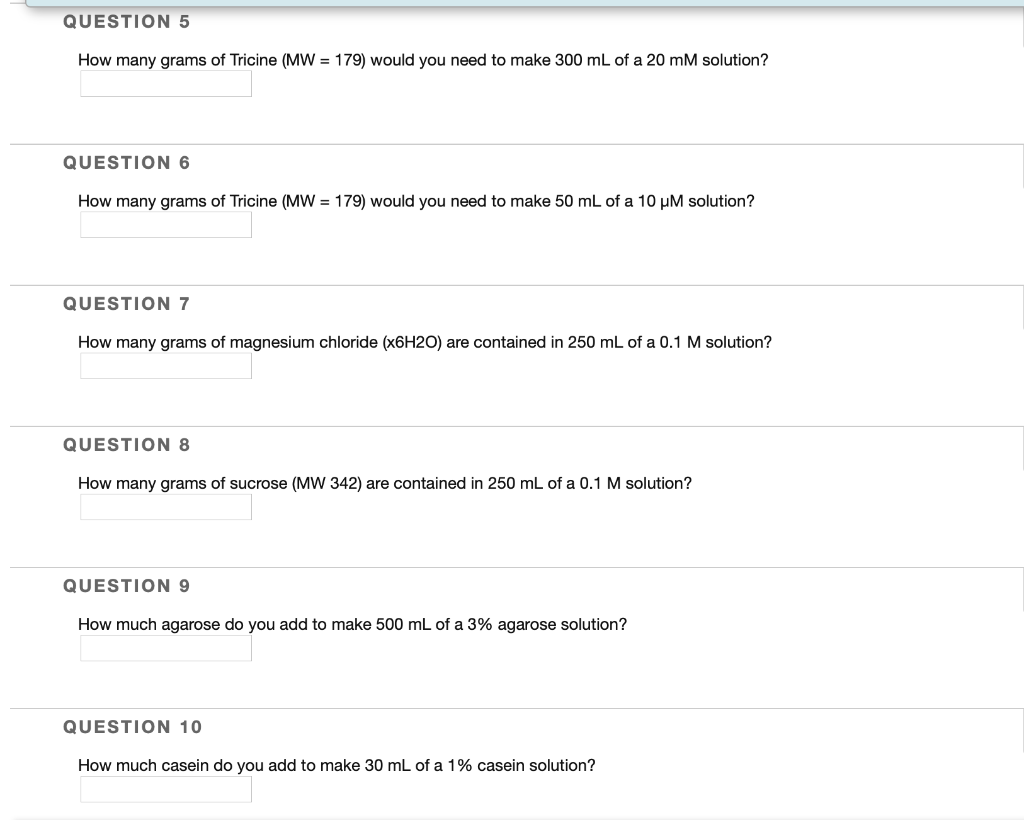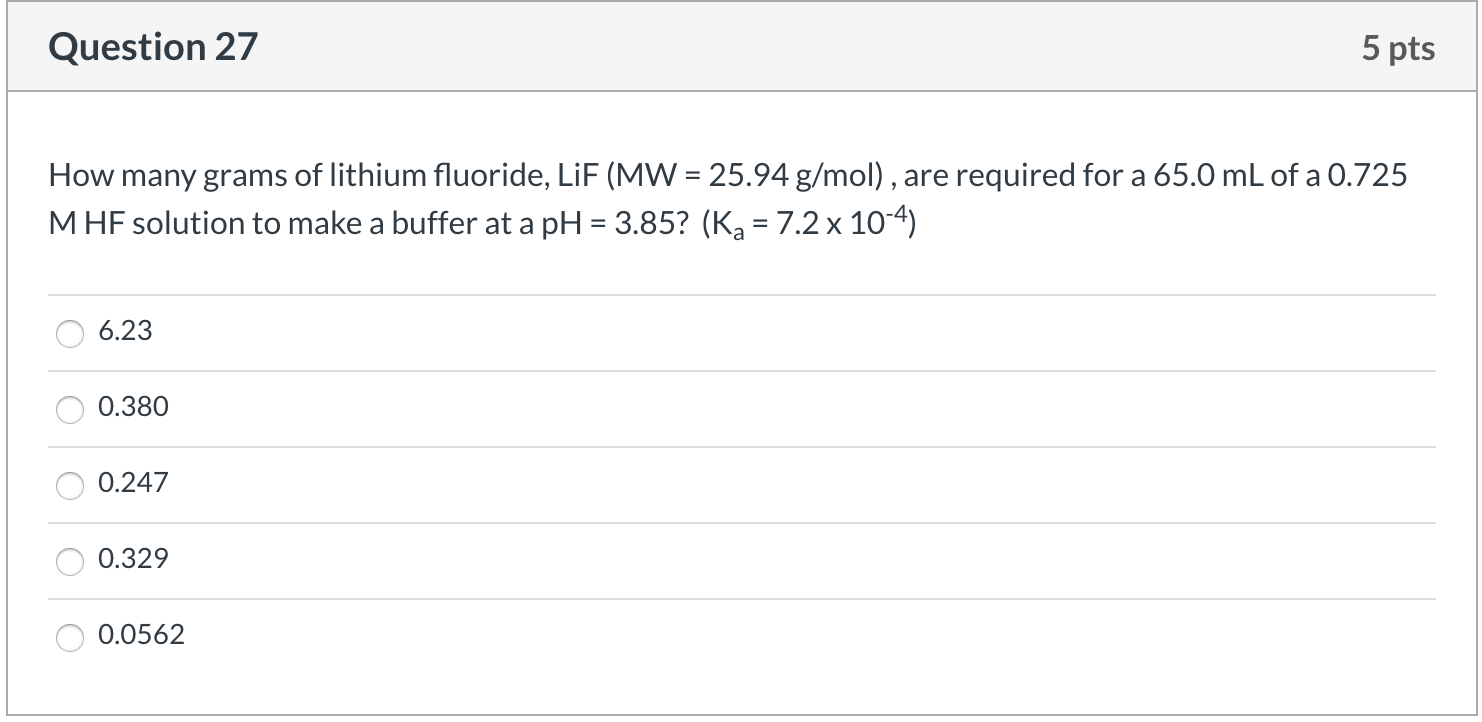Question

QUESTION 5                  8 MARKS
a) How many grams of BaCl2.2H2O (MW = 244.27 g/mol) you need to prepare 250
mL of 0.15 M chloride ion solution?
2 marks
b) It was established that this reagent was contaminated by CaCl2 (MW = 181.82
g). The content of calcium in the solid sample was found to be 4750 ppm (w/w).
What was the percentage decrease in the molar Barium ion concentration
caused by this contamination?
6 marks

hi chemists I recieved this question after my practical today to work on at home can someone please help me

How many grams of BaCl2.2H2O (MW = 244.27 g/mol) you need to prepare 250
mL of 0.15 M chloride ion solution?

M = n solute / V solution L

M = (mass / molar mass) / V solution L
MV = mass / molar mass
MV(molar) = mass
plugging values

for the mass.
MV(molar) = mass
(0.15 M)(0.25 L)(244.27 g/mol) = mass
9.16 g = mass ------Answer)

=======================

#### Earn Coins

Coins can be redeemed for fabulous gifts.

Similar Homework Help Questions
• ### how do you do this question An aqueous solution of calcium chloride contains 2 grams of calcium chloride ( molar mass 110.98 g mol-1) in 100 ml calculate the concentration of chloride ion (Cl-) in the solution

how do you do this question An aqueous solution of calcium chloride contains 2 grams of calcium chloride ( molar mass 110.98 g mol-1) in 100 ml calculate the concentration of chloride ion (Cl-) in the solution

• ### QUESTION 5 How many grams of Tricine (MW = 179) would you need to make 300...QUESTION 5 How many grams of Tricine (MW = 179) would you need to make 300 mL of a 20 mM solution? QUESTION 6 How many grams of Tricine (MW = 179) would you need to make 50 mL of a 10 UM solution? QUESTION 7 How many grams of magnesium chloride (x6H2O) are contained in 250 mL of a 0.1 M solution? QUESTION 8 How many grams of sucrose (MW 342) are contained in 250 mL of a 0.1...

• ### Question 27 5 pts How many grams of lithium fluoride, LiF (MW = 25.94 g/mol), are...Question 27 5 pts How many grams of lithium fluoride, LiF (MW = 25.94 g/mol), are required for a 65.0 mL of a 0.725 MHF solution to make a buffer at a pH = 3.85? (Ka = 7.2 x 10-4) O 6.23 O 0.380 O 0.247 O 0.329 0 0.0562